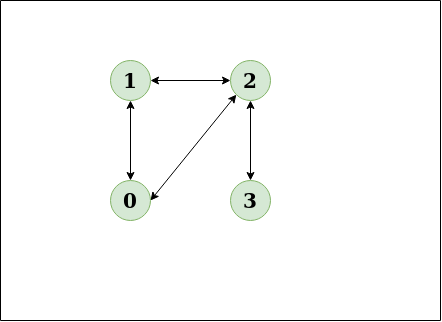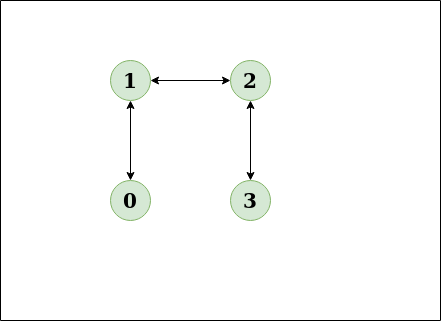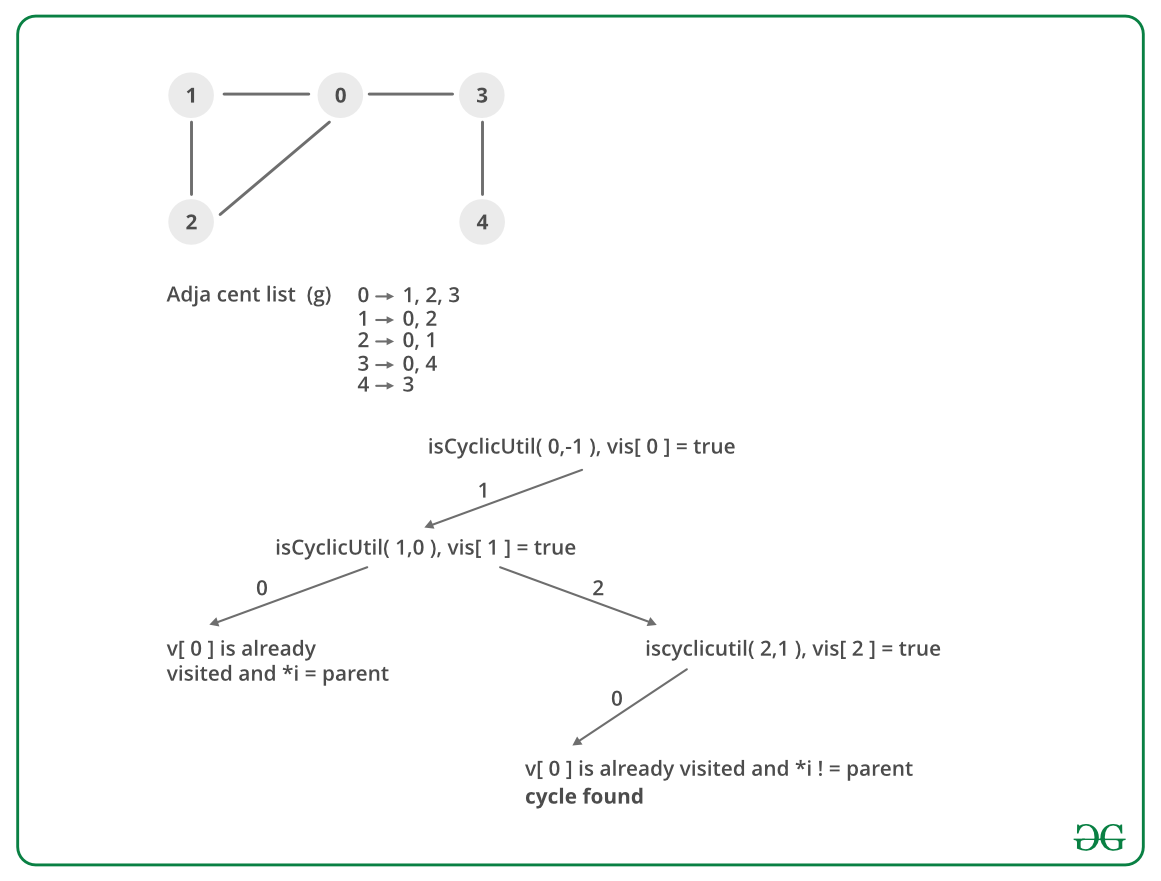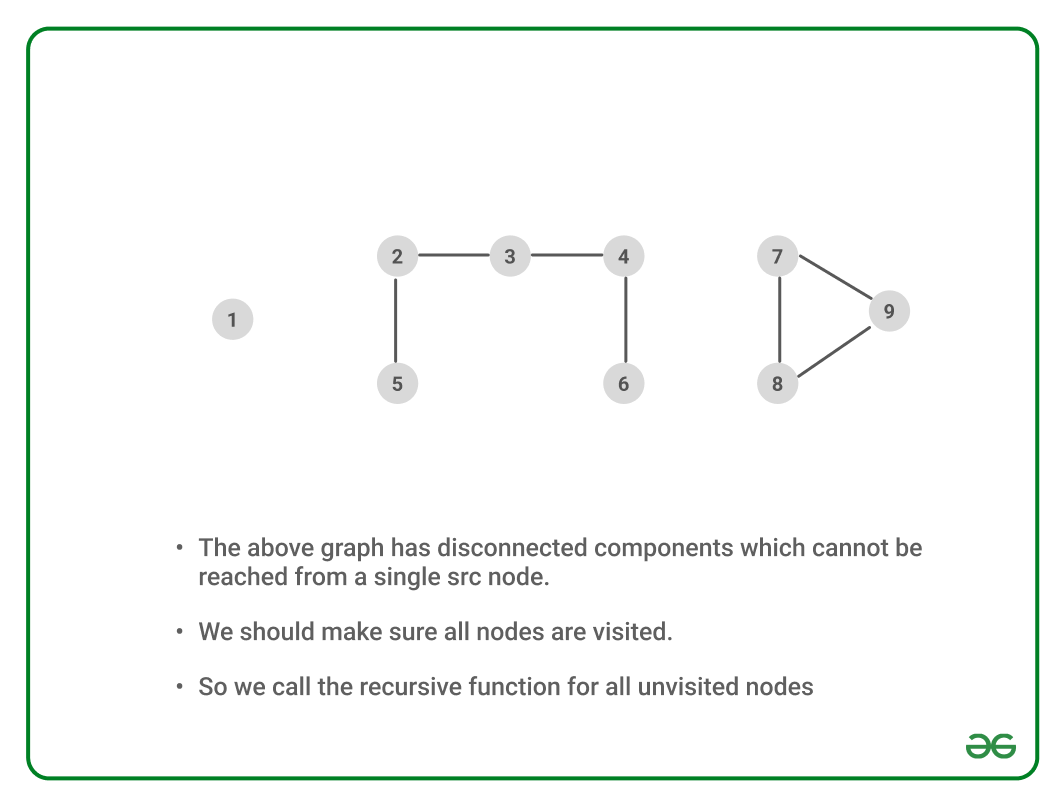Open in App
Not now

# Detect cycle in an undirected graph

• Difficulty Level : Medium
• Last Updated : 02 Feb, 2023

Given an undirected graph, The task is to check if there is a cycle in the given graph.

Example:

Input: N = 4, E = 4Output: Yes
Explanation: The diagram clearly shows a cycle 0 to 2 to 1 to 0

Input: N = 4, E = 3 , 0 1, 1 2, 2 3Output: No
Explanation: There is no cycle in the given graph

## Find cycle in undirected Graph using DFS:

Use DFS from every unvisited node. Depth First Traversal can be used to detect a cycle in a Graph. There is a cycle in a graph only if there is a back edge present in the graph. A back edge is an edge that is indirectly joining a node to itself (self-loop) or one of its ancestors in the tree produced by DFS.

To find the back edge to any of its ancestors keep a visited array and if there is a back edge to any visited node then there is a loop and return true.

Follow the below steps to implement the above approach:

• Iterate over all the nodes of the graph and Keep a visited array visited[] to track the visited nodes.
• Run a Depth First Traversal on the given subgraph connected to the current node and pass the parent of the current node. In each recursive
• Set visited[root] as 1.
• Iterate over all adjacent nodes of the current node in the adjacency list
• If it is not visited then run DFS on that node and return true if it returns true.
• Else if the adjacent node is visited and not the parent of the current node then return true.
• Return false.

Dry Run:Another possible scenario:

If No cycle is detected after running Depth First Traversal for every subgraph the there exists no cycle as shown belowGraph with disconnected components

Below is the implementation of the above approach:

## C++

 `// A C++ Program to detect` `// cycle in an undirected graph` `#include ` `#include ` `#include ` `using` `namespace` `std;`   `// Class for an undirected graph` `class` `Graph {`   `    ``// No. of vertices` `    ``int` `V;`   `    ``// Pointer to an array` `    ``// containing adjacency lists` `    ``list<``int``>* adj;` `    ``bool` `isCyclicUtil(``int` `v, ``bool` `visited[], ``int` `parent);`   `public``:` `    ``// Constructor` `    ``Graph(``int` `V);`   `    ``// To add an edge to graph` `    ``void` `addEdge(``int` `v, ``int` `w);`   `    ``// Returns true if there is a cycle` `    ``bool` `isCyclic();` `};`   `Graph::Graph(``int` `V)` `{` `    ``this``->V = V;` `    ``adj = ``new` `list<``int``>[V];` `}`   `void` `Graph::addEdge(``int` `v, ``int` `w)` `{`   `    ``// Add w to v’s list.` `    ``adj[v].push_back(w);`   `    ``// Add v to w’s list.` `    ``adj[w].push_back(v);` `}`   `// A recursive function that` `// uses visited[] and parent to detect` `// cycle in subgraph reachable` `// from vertex v.` `bool` `Graph::isCyclicUtil(``int` `v, ``bool` `visited[], ``int` `parent)` `{`   `    ``// Mark the current node as visited` `    ``visited[v] = ``true``;`   `    ``// Recur for all the vertices` `    ``// adjacent to this vertex` `    ``list<``int``>::iterator i;` `    ``for` `(i = adj[v].begin(); i != adj[v].end(); ++i) {`   `        ``// If an adjacent vertex is not visited,` `        ``// then recur for that adjacent` `        ``if` `(!visited[*i]) {` `            ``if` `(isCyclicUtil(*i, visited, v))` `                ``return` `true``;` `        ``}`   `        ``// If an adjacent vertex is visited and` `        ``// is not parent of current vertex,` `        ``// then there exists a cycle in the graph.` `        ``else` `if` `(*i != parent)` `            ``return` `true``;` `    ``}` `    ``return` `false``;` `}`   `// Returns true if the graph contains` `// a cycle, else false.` `bool` `Graph::isCyclic()` `{`   `    ``// Mark all the vertices as not` `    ``// visited and not part of recursion` `    ``// stack` `    ``bool``* visited = ``new` `bool``[V];` `    ``for` `(``int` `i = 0; i < V; i++)` `        ``visited[i] = ``false``;`   `    ``// Call the recursive helper` `    ``// function to detect cycle in different` `    ``// DFS trees` `    ``for` `(``int` `u = 0; u < V; u++) {`   `        ``// Don't recur for u if` `        ``// it is already visited` `        ``if` `(!visited[u])` `            ``if` `(isCyclicUtil(u, visited, -1))` `                ``return` `true``;` `    ``}` `    ``return` `false``;` `}`   `// Driver program to test above functions` `int` `main()` `{` `    ``Graph g1(5);` `    ``g1.addEdge(1, 0);` `    ``g1.addEdge(0, 2);` `    ``g1.addEdge(2, 1);` `    ``g1.addEdge(0, 3);` `    ``g1.addEdge(3, 4);` `    ``g1.isCyclic() ? cout << ``"Graph contains cycle\n"` `                  ``: cout << ``"Graph doesn't contain cycle\n"``;`   `    ``Graph g2(3);` `    ``g2.addEdge(0, 1);` `    ``g2.addEdge(1, 2);` `    ``g2.isCyclic() ? cout << ``"Graph contains cycle\n"` `                  ``: cout << ``"Graph doesn't contain cycle\n"``;`   `    ``return` `0;` `}`

## Java

 `// A Java Program to detect cycle in an undirected graph` `import` `java.io.*;` `import` `java.util.*;` `@SuppressWarnings``(``"unchecked"``)` `// This class represents a` `// directed graph using adjacency list` `// representation` `class` `Graph {`   `    ``// No. of vertices` `    ``private` `int` `V;`   `    ``// Adjacency List Representation` `    ``private` `LinkedList adj[];`   `    ``// Constructor` `    ``Graph(``int` `v)` `    ``{` `        ``V = v;` `        ``adj = ``new` `LinkedList[v];` `        ``for` `(``int` `i = ``0``; i < v; ++i)` `            ``adj[i] = ``new` `LinkedList();` `    ``}`   `    ``// Function to add an edge` `    ``// into the graph` `    ``void` `addEdge(``int` `v, ``int` `w)` `    ``{` `        ``adj[v].add(w);` `        ``adj[w].add(v);` `    ``}`   `    ``// A recursive function that` `    ``// uses visited[] and parent to detect` `    ``// cycle in subgraph reachable` `    ``// from vertex v.` `    ``Boolean isCyclicUtil(``int` `v, Boolean visited[],` `                         ``int` `parent)` `    ``{` `        ``// Mark the current node as visited` `        ``visited[v] = ``true``;` `        ``Integer i;`   `        ``// Recur for all the vertices` `        ``// adjacent to this vertex` `        ``Iterator it = adj[v].iterator();` `        ``while` `(it.hasNext()) {` `            ``i = it.next();`   `            ``// If an adjacent is not` `            ``// visited, then recur for that` `            ``// adjacent` `            ``if` `(!visited[i]) {` `                ``if` `(isCyclicUtil(i, visited, v))` `                    ``return` `true``;` `            ``}`   `            ``// If an adjacent is visited` `            ``// and not parent of current` `            ``// vertex, then there is a cycle.` `            ``else` `if` `(i != parent)` `                ``return` `true``;` `        ``}` `        ``return` `false``;` `    ``}`   `    ``// Returns true if the graph` `    ``// contains a cycle, else false.` `    ``Boolean isCyclic()` `    ``{`   `        ``// Mark all the vertices as` `        ``// not visited and not part of` `        ``// recursion stack` `        ``Boolean visited[] = ``new` `Boolean[V];` `        ``for` `(``int` `i = ``0``; i < V; i++)` `            ``visited[i] = ``false``;`   `        ``// Call the recursive helper` `        ``// function to detect cycle in` `        ``// different DFS trees` `        ``for` `(``int` `u = ``0``; u < V; u++) {`   `            ``// Don't recur for u if already visited` `            ``if` `(!visited[u])` `                ``if` `(isCyclicUtil(u, visited, -``1``))` `                    ``return` `true``;` `        ``}`   `        ``return` `false``;` `    ``}`   `    ``// Driver method to test above methods` `    ``public` `static` `void` `main(String args[])` `    ``{`   `        ``// Create a graph given` `        ``// in the above diagram` `        ``Graph g1 = ``new` `Graph(``5``);` `        ``g1.addEdge(``1``, ``0``);` `        ``g1.addEdge(``0``, ``2``);` `        ``g1.addEdge(``2``, ``1``);` `        ``g1.addEdge(``0``, ``3``);` `        ``g1.addEdge(``3``, ``4``);` `        ``if` `(g1.isCyclic())` `            ``System.out.println(``"Graph contains cycle"``);` `        ``else` `            ``System.out.println(``"Graph doesn't contain cycle"``);`   `        ``Graph g2 = ``new` `Graph(``3``);` `        ``g2.addEdge(``0``, ``1``);` `        ``g2.addEdge(``1``, ``2``);` `        ``if` `(g2.isCyclic())` `            ``System.out.println(``"Graph contains cycle"``);` `        ``else` `            ``System.out.println(``"Graph doesn't contain cycle"``);` `    ``}` `}` `// This code is contributed by Aakash Hasija`

## Python3

 `# Python Program to detect cycle in an undirected graph` `from` `collections ``import` `defaultdict`   `# This class represents a undirected` `# graph using adjacency list representation`     `class` `Graph:`   `    ``def` `__init__(``self``, vertices):`   `        ``# No. of vertices` `        ``self``.V ``=` `vertices  ``# No. of vertices`   `        ``# Default dictionary to store graph` `        ``self``.graph ``=` `defaultdict(``list``)`   `    ``# Function to add an edge to graph` `    ``def` `addEdge(``self``, v, w):`   `        ``# Add w to v_s list` `        ``self``.graph[v].append(w)`   `        ``# Add v to w_s list` `        ``self``.graph[w].append(v)`   `    ``# A recursive function that uses` `    ``# visited[] and parent to detect` `    ``# cycle in subgraph reachable from vertex v.` `    ``def` `isCyclicUtil(``self``, v, visited, parent):`   `        ``# Mark the current node as visited` `        ``visited[v] ``=` `True`   `        ``# Recur for all the vertices` `        ``# adjacent to this vertex` `        ``for` `i ``in` `self``.graph[v]:`   `            ``# If the node is not` `            ``# visited then recurse on it` `            ``if` `visited[i] ``=``=` `False``:` `                ``if``(``self``.isCyclicUtil(i, visited, v)):` `                    ``return` `True` `            ``# If an adjacent vertex is` `            ``# visited and not parent` `            ``# of current vertex,` `            ``# then there is a cycle` `            ``elif` `parent !``=` `i:` `                ``return` `True`   `        ``return` `False`   `    ``# Returns true if the graph` `    ``# contains a cycle, else false.`   `    ``def` `isCyclic(``self``):`   `        ``# Mark all the vertices` `        ``# as not visited` `        ``visited ``=` `[``False``]``*``(``self``.V)`   `        ``# Call the recursive helper` `        ``# function to detect cycle in different` `        ``# DFS trees` `        ``for` `i ``in` `range``(``self``.V):`   `            ``# Don't recur for u if it` `            ``# is already visited` `            ``if` `visited[i] ``=``=` `False``:` `                ``if``(``self``.isCyclicUtil` `                   ``(i, visited, ``-``1``)) ``=``=` `True``:` `                    ``return` `True`   `        ``return` `False`     `# Create a graph given in the above diagram` `g ``=` `Graph(``5``)` `g.addEdge(``1``, ``0``)` `g.addEdge(``1``, ``2``)` `g.addEdge(``2``, ``0``)` `g.addEdge(``0``, ``3``)` `g.addEdge(``3``, ``4``)`   `if` `g.isCyclic():` `    ``print``(``"Graph contains cycle"``)` `else``:` `    ``print``(``"Graph doesn't contain cycle "``)` `g1 ``=` `Graph(``3``)` `g1.addEdge(``0``, ``1``)` `g1.addEdge(``1``, ``2``)`     `if` `g1.isCyclic():` `    ``print``(``"Graph contains cycle"``)` `else``:` `    ``print``(``"Graph doesn't contain cycle "``)`   `# This code is contributed by Neelam Yadav`

## C#

 `// C# Program to detect cycle in an undirected graph` `using` `System;` `using` `System.Collections.Generic;`   `// This class represents a directed graph` `// using adjacency list representation` `class` `Graph {` `    ``private` `int` `V; ``// No. of vertices`   `    ``// Adjacency List Representation` `    ``private` `List<``int``>[] adj;`   `    ``// Constructor` `    ``Graph(``int` `v)` `    ``{` `        ``V = v;` `        ``adj = ``new` `List<``int``>[ v ];` `        ``for` `(``int` `i = 0; i < v; ++i)` `            ``adj[i] = ``new` `List<``int``>();` `    ``}`   `    ``// Function to add an edge into the graph` `    ``void` `addEdge(``int` `v, ``int` `w)` `    ``{` `        ``adj[v].Add(w);` `        ``adj[w].Add(v);` `    ``}`   `    ``// A recursive function that uses visited[]` `    ``// and parent to detect cycle in subgraph` `    ``// reachable from vertex v.` `    ``Boolean isCyclicUtil(``int` `v, Boolean[] visited,` `                         ``int` `parent)` `    ``{` `        ``// Mark the current node as visited` `        ``visited[v] = ``true``;`   `        ``// Recur for all the vertices` `        ``// adjacent to this vertex` `        ``foreach``(``int` `i ``in` `adj[v])` `        ``{` `            ``// If an adjacent is not visited,` `            ``// then recur for that adjacent` `            ``if` `(!visited[i]) {` `                ``if` `(isCyclicUtil(i, visited, v))` `                    ``return` `true``;` `            ``}`   `            ``// If an adjacent is visited and` `            ``// not parent of current vertex,` `            ``// then there is a cycle.` `            ``else` `if` `(i != parent)` `                ``return` `true``;` `        ``}` `        ``return` `false``;` `    ``}`   `    ``// Returns true if the graph contains` `    ``// a cycle, else false.` `    ``Boolean isCyclic()` `    ``{` `        ``// Mark all the vertices as not visited` `        ``// and not part of recursion stack` `        ``Boolean[] visited = ``new` `Boolean[V];` `        ``for` `(``int` `i = 0; i < V; i++)` `            ``visited[i] = ``false``;`   `        ``// Call the recursive helper function` `        ``// to detect cycle in different DFS trees` `        ``for` `(``int` `u = 0; u < V; u++)`   `            ``// Don't recur for u if already visited` `            ``if` `(!visited[u])` `                ``if` `(isCyclicUtil(u, visited, -1))` `                    ``return` `true``;`   `        ``return` `false``;` `    ``}`   `    ``// Driver Code` `    ``public` `static` `void` `Main(String[] args)` `    ``{` `        ``// Create a graph given in the above diagram` `        ``Graph g1 = ``new` `Graph(5);` `        ``g1.addEdge(1, 0);` `        ``g1.addEdge(0, 2);` `        ``g1.addEdge(2, 1);` `        ``g1.addEdge(0, 3);` `        ``g1.addEdge(3, 4);` `        ``if` `(g1.isCyclic())` `            ``Console.WriteLine(``"Graph contains cycle"``);` `        ``else` `            ``Console.WriteLine(` `                ``"Graph doesn't contain cycle"``);`   `        ``Graph g2 = ``new` `Graph(3);` `        ``g2.addEdge(0, 1);` `        ``g2.addEdge(1, 2);` `        ``if` `(g2.isCyclic())` `            ``Console.WriteLine(``"Graph contains cycle"``);` `        ``else` `            ``Console.WriteLine(` `                ``"Graph doesn't contain cycle"``);` `    ``}` `}`   `// This code is contributed by PrinciRaj1992`

## Javascript

 `// javascript Program to detect cycle in an undirected graph`   `// This class represents a undirected` `// graph using adjacency list representation`   `class Graph{`   `    ``constructor(vertices){` `        `  `        ``// No. of vertices` `        ``this``.V = vertices;` `        `  `        ``// Default dictionary to store graph` `        ``this``.graph = ``new` `Array(vertices);` `        ``for``(let i = 0; i < vertices; i++){` `            ``this``.graph[i] = ``new` `Array();` `        ``}` `    ``}` `        `    `    ``// Function to add an edge to graph` `    ``addEdge(v, w){` `        `  `        ``// Add w to v_s list` `        ``this``.graph[v].push(w);`   `        ``// Add v to w_s list` `        ``this``.graph[w].push(v);      ` `    ``}`       `    ``// A recursive function that uses` `    ``// visited[] and parent to detect` `    ``// cycle in subgraph reachable from vertex v.` `    ``isCyclicUtil(v, visited, parent){` ` `  `        ``// Mark the current node as visited` `        ``visited[v] = ``true``;`   `        ``// Recur for all the vertices` `        ``// adjacent to this vertex` `        ``for``(let i = 0; i < ``this``.graph[v].length; i++){`   `            ``// If the node is not` `            ``// visited then recurse on it` `            ``if``(visited[``this``.graph[v][i]] == ``false``){` `                ``if``(``this``.isCyclicUtil(``this``.graph[v][i], visited, v) == ``true``){` `                    ``return` `true``;` `                ``}` `            ``}` `            ``// If an adjacent vertex is` `            ``// visited and not parent` `            ``// of current vertex,` `            ``// then there is a cycle` `            ``else` `if``(parent != ``this``.graph[v][i]){` `                ``return` `true``;` `            ``}` `        ``}` `        ``return` `false``;` `    ``}`       `    ``// Returns true if the graph` `    ``// contains a cycle, else false.` `    ``isCyclic(){`   `        ``// Mark all the vertices` `        ``// as not visited` `        ``let visited = ``new` `Array(``this``.V).fill(``false``);`   `        ``// Call the recursive helper` `        ``// function to detect cycle in different` `        ``// DFS trees` `        ``for``(let i = 0; i < ``this``.V; i++){` `            ``// Don't recur for u if it` `            ``// is already visited` `            ``if``(visited[i] == ``false``){` `                ``if``(``this``.isCyclicUtil(i, visited, -1) == ``true``){` `                    ``return` `true``;` `                ``}` `            ``}` `                `  `        ``}`   `        ``return` `false``;` `    ``}`   `}`   `// Create a graph given in the above diagram` `let g = ``new` `Graph(5);` `g.addEdge(1, 0);` `g.addEdge(1, 2);` `g.addEdge(2, 0);` `g.addEdge(0, 3);` `g.addEdge(3, 4);`   `if` `(g.isCyclic() == ``true``){` `    ``console.log(``"Graph contains cycle"``);` `} ` `else``{` `    ``console.log(``"Graph doesn't contain cycle "``);` `}`   `let g1 = ``new` `Graph(3);` `g1.addEdge(0, 1);` `g1.addEdge(1, 2);`     `if``(g1.isCyclic() == ``true``){` `    ``console.log(``"Graph contains cycle"``);` `}   ` `else``{` `    ``console.log(``"Graph doesn't contain cycle "``); ` `}` `    `    `// This code is contributed by Gautam goel.`

Output

```Graph contains cycle
Graph doesn't contain cycle```

Time Complexity: O(V+E), The program does a simple DFS Traversal of the graph which is represented using an adjacency list. So the time complexity is O(V+E).
Auxiliary Space: O(V), To store the visited array O(V) space is required.

Exercise: Can we use BFS to detect cycle in an undirected graph in O(V+E) time? What about directed graphs?

My Personal Notes arrow_drop_up
Related Articles